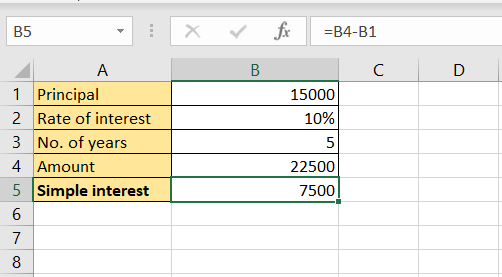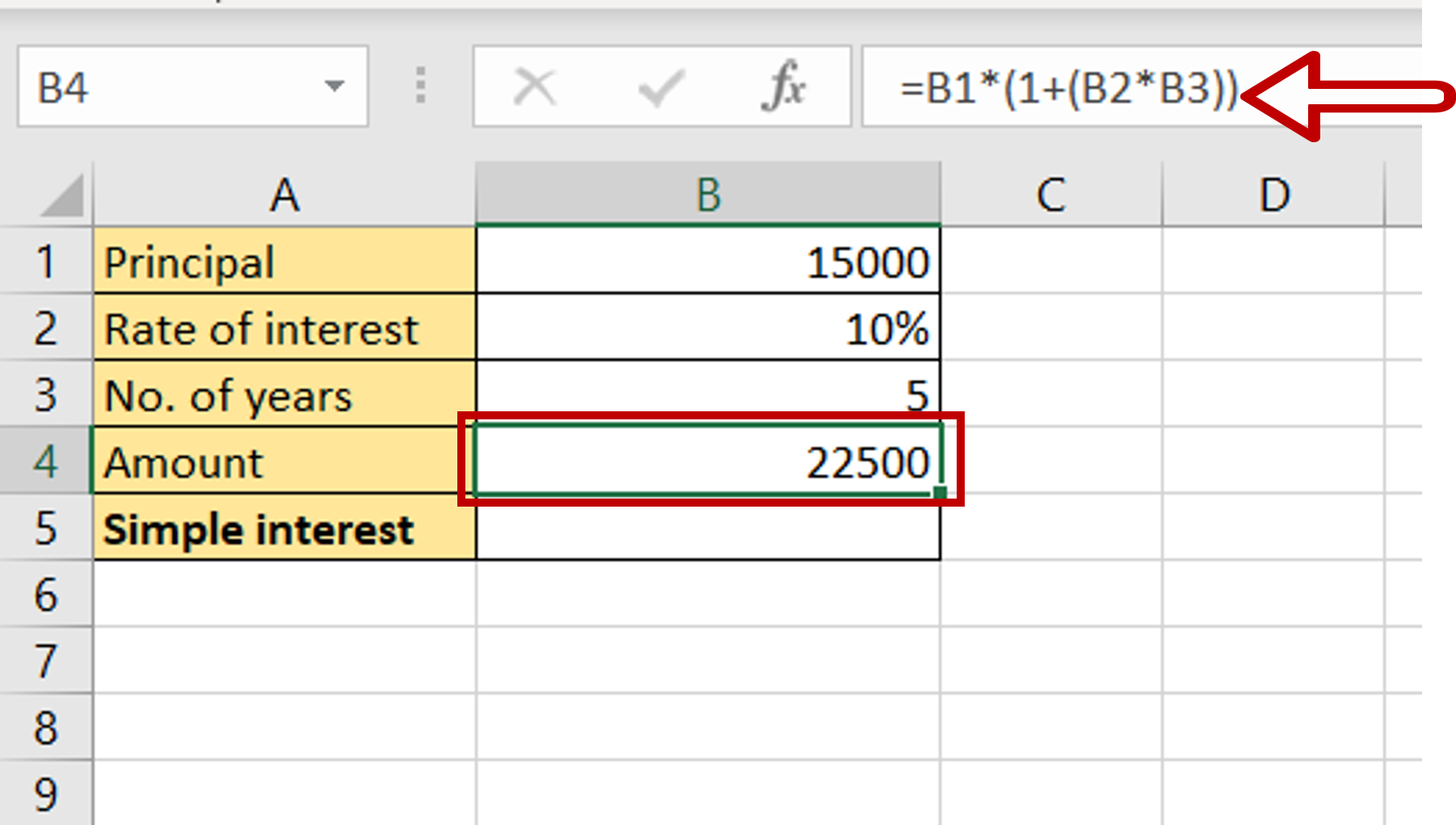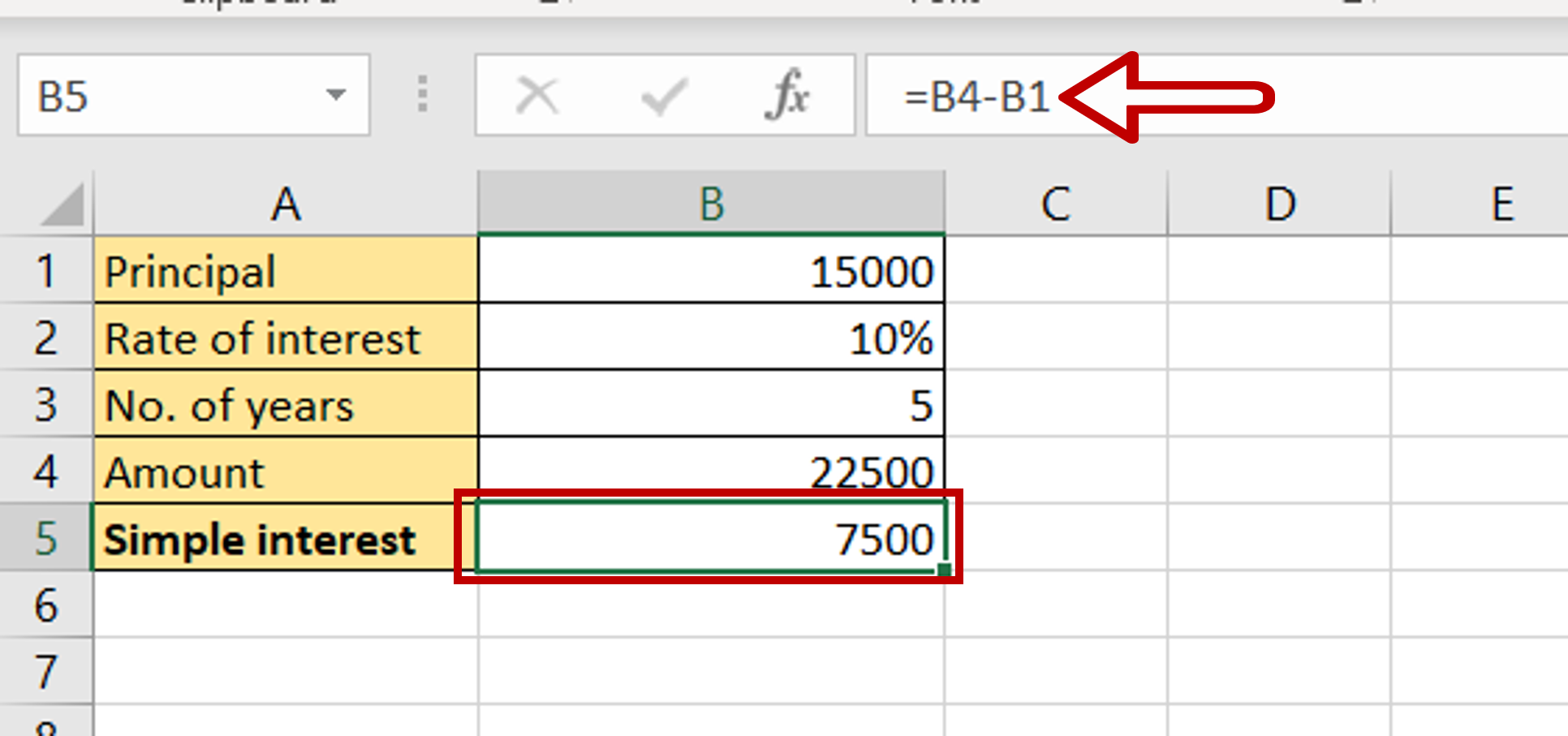# How to calculate simple interest in Excel

You can watch a video tutorial here.Excel is widely used for calculations due to the several mathematical operators and functions that it has. Many small businesses use Excel to keep track of their daily operations. Calculating simple interest can be easily done in Excel. By using cell references instead of actual values, the calculator can be used for different values. The formula is as follows:

A = P(1+rt)
A = The final amount to be paid, including the interest
P = the principal
r = the annual rate of interest
t = the number of years
Simple interest = A-P
A = The final amount to be paid, including the interest
P = the principal

### Step 1 – Compute the final amount– Select the cell in which the result is to be displayed
– Type the formula using cell references:
= Principal * (1+(Rate of interest *No. of years))
– Press Enter

### Step 2 – Compute the simple interest amount– Select the cell in which the result is to be displayed
– Type the formula using cell references:
= Amount – Principal
– Press Enter

### Step 3 – Check the result– The simple interest amount is displayed